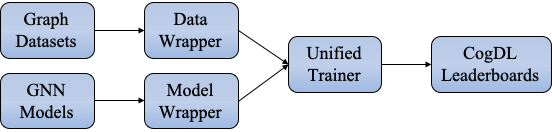# 模型训练

## 自定义模型训练逻辑

cogdl 支持选择自定义训练逻辑，“数据-模型-训练”三部分在 CogDL 中是独立的，研究者和使用者可以自定义其中任何一部分，并复用其他部分，从而提高开发效率。现在您可以使用 Cogdl 中的模型和数据集来实现您的个性化需求。

```import torch
import torch.nn as nn
import torch.nn.functional as F
from cogdl import experiment
from cogdl.datasets import build_dataset_from_name
from cogdl.layers import GCNLayer
from cogdl.models import BaseModel
class Gnn(BaseModel):
def __init__(self, in_feats, hidden_size, out_feats, dropout):
super(Gnn, self).__init__()
self.conv1 = GCNLayer(in_feats, hidden_size)
self.conv2 = GCNLayer(hidden_size, out_feats)
self.dropout = nn.Dropout(dropout)
def forward(self, graph):
graph.sym_norm()
h = graph.x
h = F.relu(self.conv1(graph, self.dropout(h)))
h = self.conv2(graph, self.dropout(h))
return F.log_softmax(h, dim=1)

if __name__ == "__main__":
dataset = build_dataset_from_name("cora")
model = Gnn(in_feats=dataset.num_features, hidden_size=64, out_feats=dataset.num_classes, dropout=0.1)
model.train()
for epoch in range(300):
out = model(dataset)
loss.backward()
optimizer.step()
model.eval()
_, pred = model(dataset).max(dim=1)
print('The accuracy rate obtained by running the experiment with the custom training logic: {:.6f}'.format(acc))
```

## 统一训练器

CogDL 为 GNN 模型提供了一个统一的训练器，它接管了训练过程的整个循环。包含大量工程代码的统一训练器可灵活实现以涵盖任意 GNN 训练设置Paper

Model

Model Wrapper

Data Wrapper

GCN

GCN

supervised

full-graph

GAT

GAT

supervised

full-graph

GraphSAGE

SAGE

sage_mw

neighbor sampling

Cluster-GCN

GCN

supervised

graph clustering

DGI

GCN

dgi_mw

full-graph

## Experiment API

CogDL在训练上提供了更易于使用的 API ，即Experiment 。我们以节点分类为例，展示如何使用 CogDL 完成使用 GNN 的工作流程。在监督设置中，节点分类旨在预测每个节点的真实标签。 CogDL 提供了丰富的通用基准数据集和 GNN 模型。一方面，您可以使用 CogDL 中的模型和数据集简单地开始运行。当您想要测试提出的 GNN 的可复现性或在不同数据集中获得基线结果时，使用Cogdl很方便。

```from cogdl import experiment
experiment(model="gcn", dataset="cora")
```

```from cogdl import experiment
from cogdl.datasets import build_dataset
from cogdl.models import build_model
from cogdl.options import get_default_args

args = get_default_args(model="gcn", dataset="cora")
dataset = build_dataset(args)
model = build_model(args)
experiment(model=model, dataset=dataset)
```

## 如何保存训练好的模型？

CogDL 支持使用 `checkpoint_path` 在命令行或 API 中保存训练的模型。例如：

```experiment(model="gcn", dataset="cora", checkpoint_path="gcn_cora.pt")
```

```experiment(model="gcn", dataset="cora", checkpoint_path="gcn_cora.pt", resume_training=True)
```

## 如何保存embeddings?

```experiment(model="prone", dataset="blogcatalog", save_emb_path="./embeddings/prone_blog.npy")
```

```experiment(
model="prone",
dataset="blogcatalog",
```# Get embedding